# H2 Math Sun 2pmMF26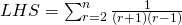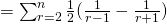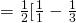Since theis a constant, the series convergences.
The sum to infinityLetdenote theterm of the AP.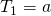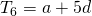Since they are consecutive terms of a GP,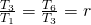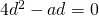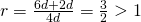, thus its not convergent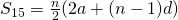Sum of first 3 terms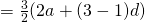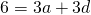—(1)
Sum of last 3 terms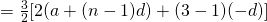; Here we consider an AP that has first termand common difference.—(2)
Sum of n terms =—(3)
Solve for n.

(i) Volume,Volume of kth later,(ii)
Since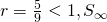exists.
Theoretical Max Volume,.
Total Volume,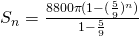We wantNot readable? Change text.